Algebra 1 : Equations / Solution Sets

Example Questions

1 2 3 4 5 6 7 8 10 Next →

Example Question #17 : Solve Problems Leading To Two Linear Equations: Ccss.Math.Content.8.Ee.C.8c

Read, but do not solve, the following problem:

Adult tickets to the zoo sell for $11; child tickets sell for$7. One day, 6,035 tickets were sold, resulting in $50,713 being raised. How many adult and child tickets were sold? Ifandstand for the number of adult and child tickets, respectively, which of the following systems of equations can be used to answer this question? Possible Answers: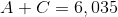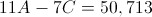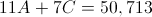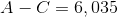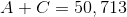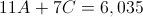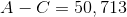Correct answer:Explanation: 6,035 total tickets were sold, and the total number of tickets is the sum of the adult and child tickets,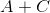. Therefore, we can say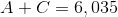. The amount of money raised from adult tickets is$11 per ticket mutiplied bytickets, or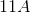dollars; similarly,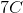dollars are raised from child tickets. Add these together to get the total amount of money raised: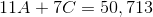These two equations form our system of equations.

Example Question #2461 : Algebra 1

A blue train leaves San Francisco at 8AM going 80 miles per hour. At the same time, a green train leaves Los Angeles, 380 miles away, going 60 miles per hour. Assuming that they are headed towards each other, when will they meet, and about how far away will they be from San Francisco?

Around 2:45AM, about 200.15 miles away from San Francisco

The two trains will never meet.

Around 3AM the next day, about 1,520 miles away from San Francisco

Around 10:43AM, about 217.12 miles away from San Francisco

Around 10:43AM, about 217.12 miles away from San Francisco

Explanation:

This system can be solved a variety of ways, including graphing. To solve algebraically, write an equation for each of the different trains. We will use y to represent the distance from San Francisco, and x to represent the time since 8AM.

The blue train travels 80 miles per hour, so it adds 80 to the distance from San Francisco every hour. Algebraically, this can be written as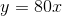.

The green train starts 380 miles away from San Francisco and subtracts distance every hour. This equation should be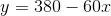.

To figure out where these trains' paths will intersect, we can set both right sides equal to each other, since the left side of each is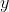.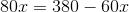add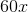to both sides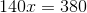divide both sides by 140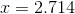Since we wrote the equation meaning time for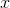, this means that the trains will cross paths after 2.714 hours have gone by. To figure out what time it will be then, figure out how many minutes are in 0.714 hours by multiplying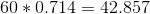. So the trains intersect after 2 hours and about 43 minutes, so at 10:43AM.

To figure out how far from San Francisco they are, figure out how many miles the blue train could have gone in 2.714 hours. In other words, plug 2.714 back into the equation, giving you an answer of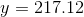.

Example Question #331 : Equations / Inequalities

We have three dogs: Joule, Newton, and Toby. Joule is three years older than twice Newton's age. Newton is Toby's age younger than eleven years. Toby is one year younger than Joules age. Find the age of each dog.

Joule: 9 years

Newton: 3 years

Toby: 8 year

Joule: 8 years

Newton: 4 years

Toby: 8 year

Joule: 5 years

Newton: Not born yet

Toby: 1 year

none of these

Joule: 12 years

Newton: 1 year

Toby: 5 year

Joule: 9 years

Newton: 3 years

Toby: 8 year

Explanation:

First, translate the problem into three equations. The statement, "Joule is three years older than twice Newton's age" is mathematically translated as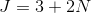where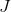represents Joule's age andis Newton's age.

The statement, "Newton is Toby's age younger than eleven years" is translated as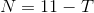where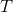is Toby's age.

The third statement, "Toby is one year younger than Joule" is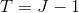.

So these are our three equations. To figure out the age of these dogs, first I will plug the third equation into the second equation. We get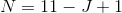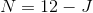Plug this equation into the first equation to get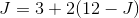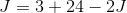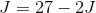Solve for. Add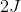to both sides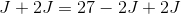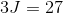Divide both sides by 3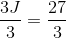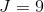So Joules is 9 years old. Plug this value into the third equation to find Toby's age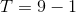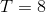Toby is 8 years old. Use this value to find Newton's age using the second equation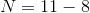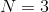Now, we have the age of the following dogs:

Joule: 9 years

Newton: 3 years

Toby: 8 years

1 2 3 4 5 6 7 8 10 Next →